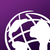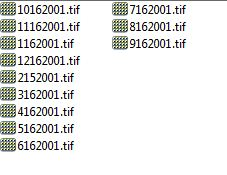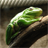# Loop through multiple folder and Calculate mean of raster of Same folder

3229
23
03-24-2017 12:18 PMOccasional Contributor III

Hi All,

I am writing a script to loop through multiple folders and calculate mean of the raster of each folder's data sets and save it in same folder. Below script i am working, but its not producing the mean rasters for each year folder, after running the script its generating only name of "mean" raster in main folder, and overlapping it .

Below images showing the name of the raster in one folder, another folder the raster name is same, only year value is changed in different year.
here how to take the year value in name of mean raster (E.g. for 2001 folder, output mean raster name would be Mean_Temp_2001.tif, E.g. for 2002 folder, output mean raster name would be Mean_Temp_2002.tif)

Below i have attached data sets also (Test_data folder)v which i am working on.

Thanks.``````import arcpy, os
from arcpy import env
arcpy.CheckOutExtension("Spatial")
arcpy.env.overwriteOutput = True
env.workspace = r"D:\Test"
outraster = env.workspace

walk = arcpy.da.Walk(env.workspace, topdown=True, datatype="RasterDataset")
for dirpath, dirnames, filenames in walk:
print dirpath
rasterList = []
for file in filenames:
raster = os.path.join(dirpath, file)
rasterList.append(raster)
rasMean = arcpy.sa.CellStatistics(rasterList,"MEAN")
rasMean.save(os.path.join(outraster,"mean.tif"))
print rasMean‍‍‍‍‍‍‍‍‍‍‍‍‍‍‍‍‍‍‍‍‍‍‍‍‍‍‍‍‍‍‍‍‍‍‍‍‍‍‍‍‍‍‍‍‍‍‍‍‍‍‍‍‍‍``````
Tags (3)
1 Solution

Accepted SolutionsbyEsri Esteemed Contributor

Hi shouvik jha , try the script below (change the input path at line 7):

``````def main():
import arcpy
import os

arcpy.CheckOutExtension("Spatial")
arcpy.env.overwriteOutput = True
ws = r'C:\GeoNet\LMFCMSF\Test_Data'

walk = arcpy.da.Walk(ws, topdown=True, datatype="RasterDataset")
for dirpath, dirnames, filenames in walk:
print "Processing folder:", dirpath

rasters = []
for filename in filenames:
raster = os.path.join(dirpath, filename)
if filename.upper().endswith('.TIF'):
if filename.upper().startswith('MEAN') == False:
rasters.append(raster)
print " - rasters found:", len(rasters)

if len(rasters) != 0:
print " - calculate mean..."
file_name_only = os.path.splitext(rasters)
tifname = file_name_only[-4:]
ras_mean = arcpy.sa.CellStatistics(rasters, "MEAN", "DATA")

ras_mean_name = os.path.join(dirpath,'Mean_{0}.tif'.format(tifname))
ras_mean.save(ras_mean_name)
print " - save mean raster as:", ras_mean_name
else:
print " - skipping folder..."

print "Finished..."

if __name__ == '__main__':
main()‍‍‍‍‍‍‍‍‍‍‍‍‍‍‍‍‍‍‍‍‍‍‍‍‍‍‍‍‍‍‍‍‍‍‍‍‍``````

This will print the following text:

``````Processing folder: C:\GeoNet\LMFCMSF\Test_Data
- rasters found: 0
- skipping folder...
Processing folder: C:\GeoNet\LMFCMSF\Test_Data\2001A
- rasters found: 12
- calculate mean...
- save mean raster as: C:\GeoNet\LMFCMSF\Test_Data\2001A\Mean_2001.tif
Processing folder: C:\GeoNet\LMFCMSF\Test_Data\2002A
- rasters found: 12
- calculate mean...
- save mean raster as: C:\GeoNet\LMFCMSF\Test_Data\2002A\Mean_2002.tif
Processing folder: C:\GeoNet\LMFCMSF\Test_Data\2003A
- rasters found: 12
- calculate mean...
- save mean raster as: C:\GeoNet\LMFCMSF\Test_Data\2003A\Mean_2003.tif
Processing folder: C:\GeoNet\LMFCMSF\Test_Data\2004A
- rasters found: 12
- calculate mean...
- save mean raster as: C:\GeoNet\LMFCMSF\Test_Data\2004A\Mean_2004.tif
Finished...‍‍‍‍‍‍‍‍‍‍‍‍‍‍‍‍‍‍‍‍``````

The resulting rasters seem to be OK...

Kind regards, Xander

23 RepliesFrequent Contributor

If you are running this as a standalone script, you will need to have arcpy check out a Spatial Analyst Extension.Occasional Contributor III

i added the line,but its behave the same as earlier .

``arcpy.CheckOutExtension("Spatial") ‍``Occasional Contributor III

@lan , i heve updated  above code, but its producing only single output name "mean" and overlapping the output for multiple time in main folder, output not save in source of input raster folderbyMVP Frequent Contributor

Take this out of the loop.

``````rasMean = arcpy.sa.CellStatistics(rasterList,"MEAN")
rasMean.save(os.path.join(outraster,"mean.tif"))
print rasMean‍‍‍``````

Assemble the rasterList first, then calculate the meanOccasional Contributor III

Thank you, May i get one example of thisbyMVP Legendary Contributor

Neil is suggesting dedenting the last 3 lines so that cell statistics is performed on the whole list rather incrementally as your script is doing.  Note, all the folders must be within one folder or a subfolder within itOccasional Contributor III

Hi, I have updated my script, Below script printing only folder name, its not calculating the mean of raster from different folder

Script:

``````import arcpy, os
from arcpy import env
arcpy.CheckOutExtension("Spatial")
arcpy.env.overwriteOutput = True
env.workspace = r"D:\Test"
outraster = env.workspace

walk = arcpy.da.Walk(env.workspace, topdown=True, datatype="RasterDataset")
for dirpath, dirnames, filenames in walk:
print dirpath
rasterList = []
for file in filenames:
raster = os.path.join(dirpath, file)
rasterList = arcpy.ListRasters("TIF")
for Rasters in rasterList:
rasMean = arcpy.sa.CellStatistics(Rasters,"MEAN", "DATA")
file_name_only = os.path.splitext(Rasters)
tifname = file_name_only[-4:]
rasMean.save(os.path.join(outraster,'Mean_{0}.tif'.format(tifname)))
print rasMean‍‍‍‍‍‍‍‍‍‍‍‍‍‍‍‍‍‍‍‍‍‍‍‍‍‍‍‍‍‍‍‍‍‍‍‍‍‍‍‍‍‍‍‍‍‍‍‍‍‍‍‍‍‍‍‍‍‍‍‍‍‍‍``````

After running the script, its printing folder only

``````D:\Test
D:\Test\2001
D:\Test\2002
D:\Test\2003
D:\Test\2004
D:\Test\2005
D:\Test\2006
D:\Test\2007
D:\Test\2008
D:\Test\2009‍‍‍‍‍‍‍‍‍‍‍‍‍‍‍‍‍‍‍‍``````byMVP Legendary Contributor

You do realize that rasmean is a raster and not a single number don't you?  And don't you want lines 16-21 indented one more level?Occasional Contributor III

Patterson i have updated the line 16-19, still script behave the same

``````for Rasters in rasterList:
rasMean = arcpy.sa.CellStatistics(Rasters,"MEAN", "DATA")
file_name_only = os.path.splitext(Rasters)
tifname = file_name_only[-4:]‍‍‍‍``````